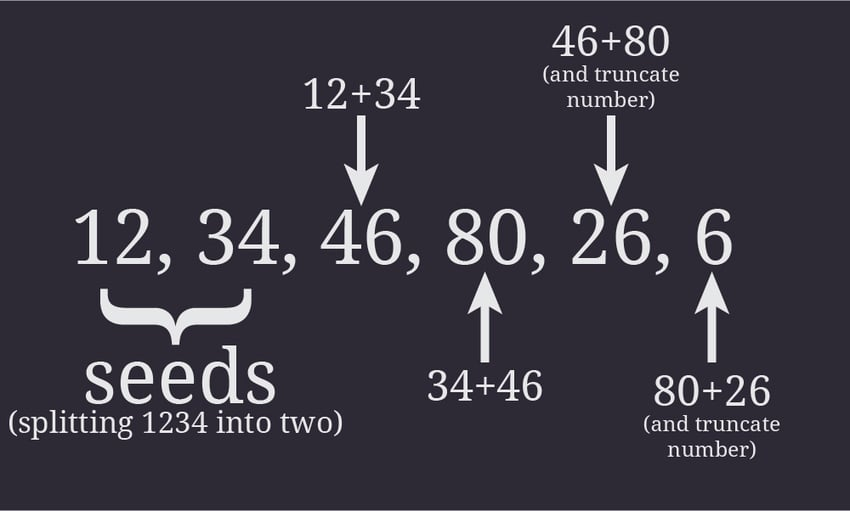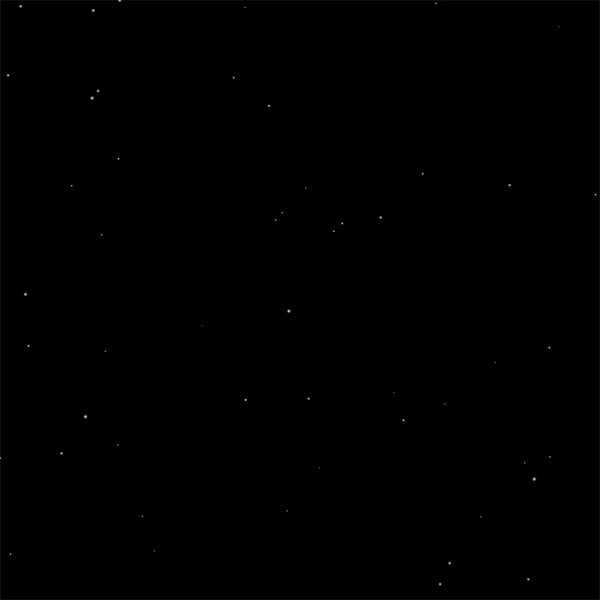# Coding a Custom Sequence Generator to Render a StarscapeIn my previous article, I explained the difference between a pseudorandom number generator and a sequence generator, and examined the advantages a sequence generator has over a PRNG. In this tutorial we'll code a fairly simple sequence generator. It generates a string of numbers, manipulates and interprets this sequence, and then uses it to draw a very simple starscape.

Note: Although this tutorial is written using Java, you should be able to use the same techniques and concepts in almost any game development environment.

## Creating and Initializing the Image

The first thing we've got to do is create the image. For this sequence generator, we're going to create a 1000×1000px image to keep number generation as simple as possible. Different languages do this differently, so use the necessary code for your dev platform.

When you've successfully created the image, it's time to give it a background color. Since we're talking about a starry sky, it would be more sensible to start with a black (#000000) backdrop and then add the white stars, rather than the other way round.

## Making a Star Profile and a Star Field

Before we start working on the sequence generator, you should figure out where you want to head with it. This means knowing what you want to create, and how different seeds and numbers vary what you want to create – in this case the stars.

To do this we need to create a sample star profile which will contain class variables that indicate some of the stars' properties. To keep things simple, we're going to start off with just three attributes:

• x-coordinate
• y-coordinate
• size

Each of the three attributes will have values ranging from 0 to 999, which means that each attribute will have three digits allocated to it. All this will be stored in a Star class.

Two important methods in the Star class are getSize() and getRadiusPx(). The getSize() method returns the star's size, scaled down to a decimal number between zero and one, and the getRadiusPx() method returns how big the star's radius should be in the final image.

I've found that 4 pixels makes for a good maximum radius in my demo, so getRadiusPx() will simply return the value of getSize() multiplied by four. For example, if the getSize() method returns a radius of 0.4, the getRadiusPx() method would give a radius of 1.6px.

We should also make a very simple class whose job is to keep track of all the stars in each sequence of stars. The Starfield class just consists of methods which add, remove or retrieve stars from an ArrayList. It should also be able to return the ArrayList.

## Planning the Sequence Generator

Now that we've finished the star profile and initialised the image, we know some important points about the sequence generator we want to create.

First of all, we know that the width and height of the image is 1000px. This means that, to exploit the resources at hand, the range of x- and y-coordinates must fall in the range 0-999. Since two of the required numbers fall in the same range, we can apply the same range to the star's size to keep uniformity. The size will then be scaled down later on when we interpret the series of numbers.

We're going to be using a number of class variables. These include: s_seed, a single integer that defines the whole sequence; s_start and s_end, two integers that are generated by splitting the seed into two; and s_current, an integer that contains the most recently generated number in the sequence.See this image from my previous article. 1234 is the seed, and 12 and 34 are the initial values of s_start and s_end.
Tip: Note that every number generated originates from the seed; there's no call to random(). This means that the same seed will always generate the same starscape.

We'll also be using s_sequence, a String which will hold the overall sequence. The last two class variables are s_image (of type Image – a class we'll create later on) and s_starfield (of type Starfield, the class we just created). The first one stores the image, while the second one contains the starfield.

The route we're going to take to create this generator is quite simple. First, we need to make a constructor which accepts a seed. When this is done, we need to create a method which accepts an integer representing the number of stars it must create. This method should then call the actual generator to come up with the numbers. And now starts the real work... creating the sequence generator.

## Coding the Sequence Generator

The first thing a sequence generator has to do is accept a seed. As mentioned, we will be splitting the seed into two: the first two digits, and the last two digits. For this reason, we must check whether the seed has four digits, and pad it with zeros if it doesn't. When this is done, we can then split the seed string into two variables: s_start and s_end. (Note that the seeds themselves won't be part of the actual sequence.)

So:

• seed = 1234 means s_start = 12 and s_end = 34
• seed = 7 means s_start = 00 and s_end = 07
• seed = 303 means s_start = 03 and s_end = 03

Next in line: create another method which, given the two numbers, generates the next number in the sequence.

Finding the right formula is a weary process. It usually means hours of trial-and-error work trying to find a sequence which doesn't involve too many patterns in the resulting image. Hence, it would be wiser to find the best formula once we can actually see the image, rather than now. What we are interested in right now is finding a formula that generates a sequence which is more or less random. For this reason, we will use the same formula used in the Fibonacci sequence: addition of the two numbers.

When this is done, we can now move on and start creating the sequence. In another method, we will be manipulating the initial seed to generate a whole stream of numbers, which can then be interpreted as the star profile's attributes.

We know that for a given star we need nine digits: the first three define the x-coordinate, the middle three define the y-coordinate, and the last three define the size. Therefore, as was the case when feeding the seed, to keep uniformity throughout it's important to make sure that each generated number has three digits. In this case, we also have to truncate the number if it is greater than 999.

This is quite similar to what we did before. We just need to store the number in a temporary String, temp, then dispose of the first digit. If the number doesn't have three digits, we should then pad it with zeros like we did earlier.

With that wrapped up, we should now make another method which creates and returns a star profile each time we generate three numbers. Using this method, we can then add the star to the ArrayList of stars.

## Putting It All Together

After this is finished, we can assemble the generator. It should accept the number of stars it has to generate.

We already know that for one star, we need nine digits, so this generator needs to keep count of the number of characters in the strings. The counter, s_counter, will store the maximum length of the sequence. Therefore we multiply the number of stars by nine and remove one since a String starts from index zero.

We also need to keep count of the number of characters we have created since we last generated a star. For this task, we are going to use s_starcounter. In a for loop, which will repeat until the series' length equals s_counter, we can now call the methods we've created so far.

Tip: We must not forget to replace s_start and s_end, or else we will keep on generating the same number over and over again!

## Drawing Stars

Now that the difficult part is over, it's finally time to move over to the Image class, and start drawing stars.

In a method which accepts a Starfield, we first create an instance of a Color, then retrieve the number of stars we must draw. In a for loop, we're going to draw all the stars. After making a copy of the current star, it's important that we retrieve the star's radius. Given that the number of pixels is an integer, we should add to the radius to make it an integer.

A radial gradient's opacity depends on the distance of a pixel from the center. The center of the circle will have coordinates (0,0). Using the most common convention, any pixel to the left of the center has a negative x-coordinate, and any pixel below has a negative y-coordinate.

Because of this, the nested for loops start with a negative number. Using Pythagoras' theorem we calculate the distance from the center of the circle and use it to retrieve the opacity. For stars which have the smallest possible radius (1px), their opacity depends solely on their size.

To wrap things up, we need to make a method which accepts a String and uses it to save the image with that file name. In the generator, we should create the image first. Then, we should call these last two methods from the sequence generator.

In the Main class, we should create an instance of the sequence generator, assign it a seed and get a good number of stars (400 should be enough). Try to run the program, fix any errors, and check the destination path to see what image was created.

## Improvements

There are still some changes we can make. For example, the first thing you would have noticed is that the stars are clustered in the center. To fix that, you would have to come up with a good formula which eliminates any patterns. Alternatively you can create a number of formulae and alternate between them using a counter. The formulae we used were these:

There is one more simple improvement we can implement. If you look up at the sky, you'll see a few big stars and many more small ones. However, in our case the number of small stars is roughly the same as the number of big stars. To fix this, we just have to return to the getSize() method in the Star class. After making the size a fraction of one, we have to raise this number to the power of an integer – for example four or five.

Running the program one last time should give you a satisfactory result.The final result – a whole starscape procedurally generated by our Sequence Generator!

## Conclusion

In this case, we used a Sequence Generator to procedurally generate a background. A Sequence Generator like this could have many more uses – for instance, a z-coordinate could be added to the star so that instead of drawing an image, the could would generate stars as objects in a 3D environment.